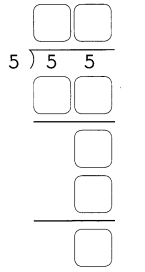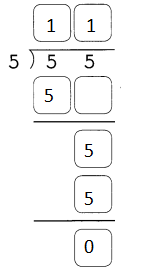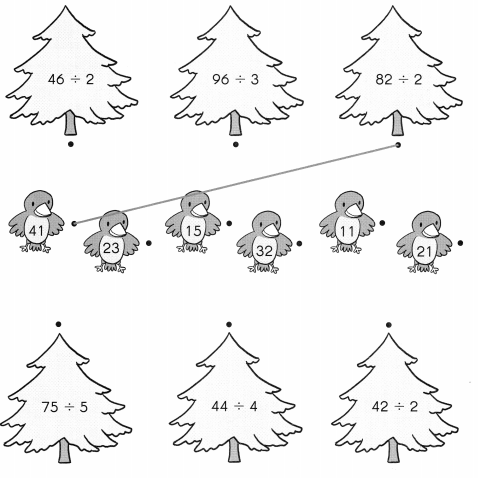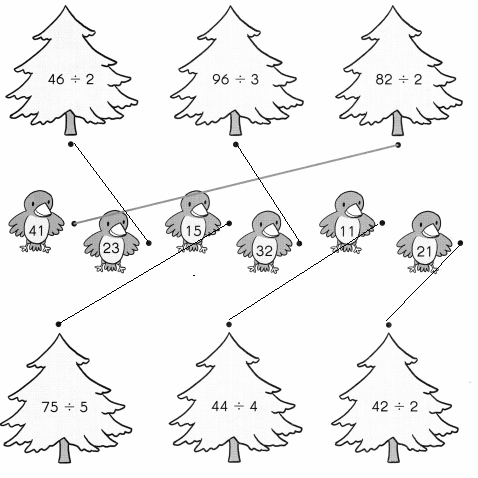# Math in Focus Grade 3 Chapter 8 Practice 4 Answer Key Division Without Remainder and Regrouping

Practice the problems of Math in Focus Grade 3 Workbook Answer Key Chapter 8 Practice 4 Division Without Remainder and Regrouping to score better marks in the exam.

## Math in Focus Grade 3 Chapter 8 Practice 4 Answer Key Division Without Remainder and Regrouping

Divide. Then match the answers to the correct pictures.

Question 1.
44 ones ÷ 4 = ___ ten ____ one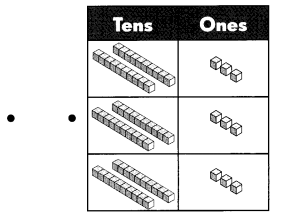44 ones ÷ 4 = 4 ten 4 one

Question 2.
69 ones ÷ 3 = ___ tens ____ ones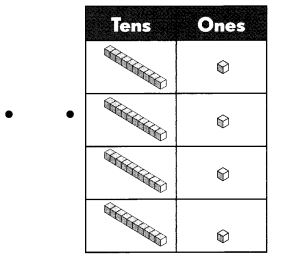69 ones ÷ 3 = 6 tens 9 ones
Explanation:
Matched the Division expression to base ten blocks table.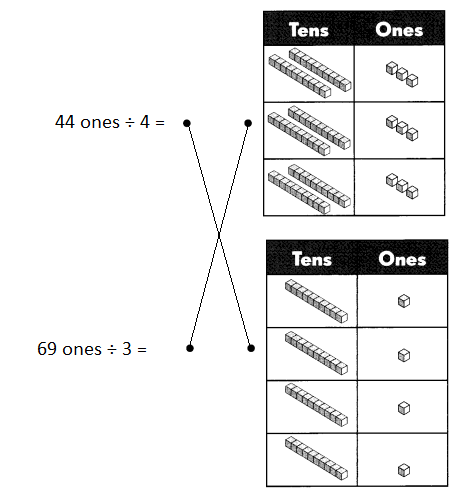Divide.

Question 3.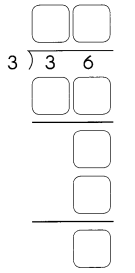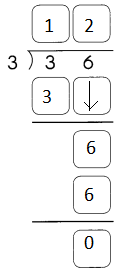Explanation:
36 ÷ 3 = 12 is the quotient and remainder is o

Question 4.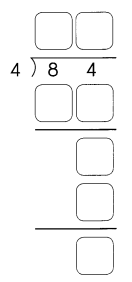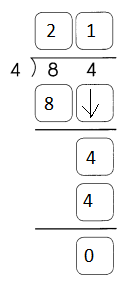Explanation:
84 ÷ 4 = 21 is the quotient and remainder is o

Question 5.